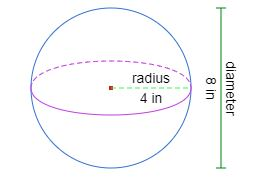# Volume of a sphere calculator

=
Your browser does not support the HTML5 canvas tag.

Input radius or diameter length to calculate the volume of a sphere.

### Volume calculators

This is a calculator that specifically calculates the volume of a sephere or a ball , support metric and imperial units (inches, feet, yards, mm, cm or meter), and volume result can convert to different unit, with calculation formula and dynamic visual sphere, it helps us to get answers and understand the results more easily.

### How to use this calculator ?

1. Choose to use the diameter or radius to calculate the volume.
2. Input the length of diameter or radius
3. Accept input decimals or fraction, eg. 3.4, 1.7, 5 1/4 or 3/5
4. Choose the unit of diameter or radius
5. Choose the unit of volume you would like to know
6. It will automatically calculate the results and react interactively.
7. The result of the calculation will be rounded.

### How to calculate the volume of a sphere ?

A sphere is a perfectly round geometrical object that is three dimensional, with every point on its surface equidistant from its center. Many commonly-used objects such as balls or globes are spheres. If you want to calculate the volume of a sphere, you just have to find its radius and plug it into a simple formula,
V = 4⁄3πr³.

### Sphere Volume Formula

The formula for the volume of a sphere is 4/3 times pi times the radius cubed. Cubing a number means multiplying it by itself three times, in this case, the radius times the radius times the radius.

V = 4⁄3πr³
πis the ratio of a circle's circumference to its diameter

### Calculation example

Find the volume of a sphere with radius 4 inches.Volume = 4⁄3π4³
4 ÷ 3 × 3.141592653589793 × 4 × 4 × 4 = 268.082573106329.
After rounding, the volume is 268.08 cubic inches.

If we want to convert units of volume into different units, we can convert the units of radius to same of volume first,
for example,
a sphere with radius of 9 inches.
What is it's volume in ft³?

radius 9 in = 9 ÷ 12 ft = 0.75 ft Volume = 4⁄3π0.75³
4 ÷ 3 × 3.141592653589793 × 0.75 × 0.75 × 0.75 = 1.7671458676442584
after rounding, the volume is 1.77 cubic feet.

If we only have the number of diameter, half the diameter is the radius, just divide the diameter by 2, and we will have the radius.